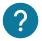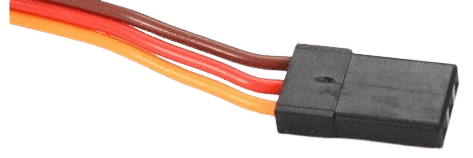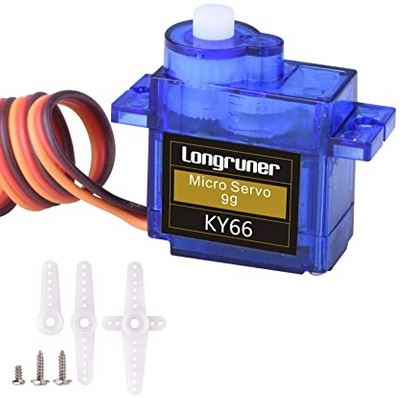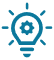# What is a servomotor? How to use it in your project?

## Introduction

A servomotor is a motor capable to stay in a position given by the user. A servomotor can’t exceed a position of 180°. According to the servomotor you have choosen, you can have a tork important to stay at the position choosen.

All theses features make this motor a useful component to open a door, open a valve or the direction of the car.

Nevertheless if you want to spin a propeller or to use the motor as a dynamo, you will need a motor dc.

If you want a motor which keep the position and turn at 360 degrees, you can use a stepper motor.

In this course we will see how to control your servomotor by two ways: a program which turn the servomotor from 0° to 180° and go back to the initial position and a program to control the servomotor by yourself with the serial monitor.### How it works?

A servomotor is composed of different elements:

• A small dc motor
• A reducer which reducing the speed of the dc motor and increase the tork to have more power to stay in the position.
• A potentiometer to generate a variable voltage proportionate at the angle of the output axis.The servomotor is controlled with pulse width modulation (pwm) with a fixed frequency of 50 hz (T=20 ms) and the high state of the signal determines the position of the servomotor.That’s mean that the duration of the pulse determinates the absolut angles therefore the position of the servomotor.

This signal is repeated periodically, in general every 20 milliseconds, which permits at the card to control and correct the continuously the position. This one is measured by the potentiometer.

The servomotor works with three wires:

• Red wire: the power supply between (3.3V and 5V).
• Black wire: The ground (0V).
• Yellow wire: the input of the signal.### Which one to choose?

If you want to use a servomotor to do Arduino, we advise you to use the MG995 or the SG90. Theses both servomotors are small and a torque of 25kg, which convenient for Arduino’s projects.Nevertheless if you need a servomotor with a torque more important, you can choose 35kg servomotors.### A) Turn your servomotor from 0° to 180°

In this part we will see how to turn your servomotor from 0° to 180° and back to the initial position.``````#include <Servo.h>

Servo myServomotor;  // On create an object servomotor
int pos = 0;    // variable to store the position

void setup() {
myServomotor.attach(9);  // We attach the servomotor to the pin 9. On attache le servomoteur a la broche 9
}

void loop() {
for (pos = 0; pos <= 180; pos += 1) { // Loop from 0 to 180 degrees
myServomotor.write(pos);              // We give the position to the servomotor
delay(15);                       // We make a break of 15 millisecondes
}
for (pos = 180; pos >= 0; pos -= 1) { // Loop from 180 to 0 degrees
myServomotor.write(pos);              // We give the position to the servomotor
delay(15);                       // We make a break of 15 millisecondes
}
}
``````

### B) Control your servomotor with the serial monitor

This is the program to control the servomotor with the serial monitor. The circuit remains the same.

``````#include <Servo.h>
Servo myServomotor;
int position_question;

void setup(){
Serial.begin(9600);
myServomotor.attach(9);
Serial.println("-------------------------");
Serial.println(" Give the position of the servomotor between 0 and 180 degrees :");
}

void loop() {
if (Serial.available()) {
Serial.println("-------------------------");
Serial.print(" Give the position of the servomotor between 0 and 180 degrees :");
position_question = Serial.parseInt();
Serial.println(position_question);
}
if (position_question < 0) {
Serial.println(" The value is lower than 0, therefore 0 will be taken");
position_question=0;
}

if (position_question > 180) {
Serial.println(" The value is higher than 180, therefore 180 will be taken");
position_question=180;
}
myServomotor.write(position_question);
}

``````
This is what we can see on the serial monitor:## Control the servomotor with a potentiometer

We will see now how to control a servomotor with a potentiometer. This project has a lot of applications possible, such as control a robot,a mechanical arm or even open a door.``````#include <Servo.h>
int Potentiometer; // Variable for the potentiometer
int Conversion;
Servo myServomotor;  // We creat an object servomotor

void setup() {
myServomotor.attach(9);  // We attach the servomotor to the pin 9
}

void loop() {
Conversion=map(Potentiometer,0,1023,180,0); // We convert this value to range from 0° to 180°
myServomotor.write(Conversion);              // We give the position to the servomotor
}

``````

## How to write the program without the library?

The servomotor works with a library which will simplify its use.

In this part we will show the different functions contains in the library if you want to directly include it in your program.

attach(pin) : Function to associate the servomotor to the pin of the Arduino card which is plugged in.

Here is the program corresponding to attach(pin) function:

``````uint8_t Servo::attach(int pin, int min, int max)
{
if(this->servoIndex < MAX_SERVOS ) { // If the number of servomotor is lower than the maximum of servomotor that we can controled (12)
pinMode( pin, OUTPUT) ; // We associate the servomotor to the output pin
servos[this->servoIndex].Pin.nbr = pin;

this->min  = (MIN_PULSE_WIDTH - min)/4; // We define the minimum position of the servomotor
this->max  = (MAX_PULSE_WIDTH - max)/4; // We define the maximum position of the servomotor
// On initialise le timer
timer16_Sequence_t timer = SERVO_INDEX_TO_TIMER(servoIndex);
if(isTimerActive(timer) == false)
initISR(timer);
servos[this->servoIndex].Pin.isActive = true;  // We activate the pin after the timer
}
return this->servoIndex ;
}
``````
• write(angle): To indicate to the servomotor which angle its need to move.

Here is the program corresponding to the function write(angle) in the library:

``````void Servo::write(int value) {
if(value < MIN_PULSE_WIDTH) {
if(value < 0) value = 0; //If the value is lower than 0 degree, we assign 0 to value
if(value > 180) value = 180; // If the value is higher to 180 degres, we assign 180 to the value
value = map(value, 0, 180, SERVO_MIN(),  SERVO_MAX());
}
this->writeMicroseconds(value); // We write the value with the function writeMicroseconds.
}``````
• read(pin): Its indicate the angle in which the servomotor is placed.

Voici le code correspondant à la fonction read(pin) dans la librairie :

``````int Servo::read() // return the actual position of the servomotor
{
return  map( this->readMicroseconds()+1, SERVO_MIN(), SERVO_MAX(), 0, 180);
}
``````
• attached: Here is the function to attach the servomotor to the pin whose plugged in.

Here is the program corresponding to the writeMicroseconds function in the library:

``````bool Servo::attached() // Return the associated pin at the servomotor
return servos[this->servoIndex].Pin.isActive ;
}``````
• writeMicroseconds: To adjust extreme positions

This program correspond to the function writeMicroseconds in the library:

``````void Servo::writeMicroseconds(int value){
// This funciton is used to create the link between the servomotor and the program
byte channel = this->servoIndex;
if( (channel < MAX_SERVOS) )   // ensure channel is valid
{
if( value < SERVO_MIN() )          // We verify if the values are correct
value = SERVO_MIN();
else if( value > SERVO_MAX() )
value = SERVO_MAX();

value = value - TRIM_DURATION;
value = usToTicks(value);
uint8_t oldSREG = SREG;
cli();
servos[channel].ticks = value;
SREG = oldSREG;
}
}``````

## To go further: Control your servomotor with python